International Journal of Geomagnetism and Aeronomy
Vol. 2, No. 3, December 2001

# On the atmospheric response to solar-particle events

K. O'Brien

Northern Arizona University, Flagstaff, Arizona

H. H. Sauer

### Abstract

High-energy solar particles, produced in association with solar flares and coronal mass ejections, occasionally bombard the Earth's atmosphere, resulting in radiation intensities additional to the already-present cosmic radiation. Access of these particles to the Earth's vicinity during times of geomagnetic disturbance are not adequately described by using static geomagnetic field models. These solar fluxes are also often distributed non-uniformly in space, so fluxes measured by satellites at great distances from the Earth and which sample large volumes of space around the Earth may not accurately predict fluxes locally at the Earth's surface. We present here a method that uses the ground level neutron monitor counting rates as adjoint sources of the flux in the atmosphere immediately above them to obtain solar-particle ionization rates as a function of position over the Earth's surface. We have applied this approach to the large September 29-30, 1989, event ground level (GLE 42) to obtain the magnitude and distribution of the solar-particle ionization from an atypically large event. The results of these calculations clearly show the effect of the softer particle spectra associated with solar particle events, as compared with cosmic rays, resulting in a greater sensitivity to the geomagnetic field, and, unlike cosmic rays, the absence of a "knee" near 60o geomagnetic latitude.

### 1. Introduction

Solar flares and coronal mass ejections (CMEs) can accelerate hydrogen (and some helium and heavier nuclei) ions to high energies by means of the shock-acceleration mechanism.

The strong shocks driven by the faster CMEs are effective in accelerating a small fraction of the coronal and solar wind they intercept to very high energies. The intense energetic particle events produced by CME-driven shocks typically last for several days or longer and are found in CMEs originating from virtually anywhere on the visible solar disk. It is now well established that most major energetic proton events observed in the vicinity of the Earth are the result of particle acceleration occurring in the outer corona and in interplanetary space at shocks driven by fast CMEs [Gosling, 1997]. The September 29-30 ground level event (GLE 42), which satisfies these criteria, was undoubtedly the result of CME shock acceleration.

When the Earth is intercepted by a shock or other interplanetary disturbance, the induced current in the magnetosphere affects the geomagnetic field in a complex manner, changing the distribution of cutoff rigidities and usually reducing them.We have used a modification of Ellison-Ramaty's [Ellison and Ramaty, 1985] acceleration theory to describe the spectrum resulting from shock acceleration:

y(t) = ap-g

p=(E2 + 2mE)1/2

where y(t) is the incident proton angular flux per MeV c-1 cm-2 s-1 sr-1 as a function of time, a =a(t) is a proportionality constant, p is the momentum in MeV c-1, g= g(t) is the index, or exponent, of the momentum, m is the proton mass, and E is the proton kinetic energy in MeV (compare Figure 1).

By multiplying y by the appropriate Jacobian j, the flux per unit energy, rather than per unit momentum, may be obtained:

j = y[p/(E +m)]

If the Earth is in the right position, it may be intercepted by the plasma accelerated by a prior shock. This plasma will affect the geomagnetic field in a complex manner, changing the distribution of cutoff rigidities and usually reducing them.

Rigidity is the momentum of a particle divided by its charge. An alpha particle with the same momentum per nucleon as a proton will have twice its rigidity per nucleon, as half the nucleons are uncharged.

Charged particles experience a V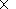B force as they propagate through the Earth's magnetic field which causes them to continuously change course, to bend through a complicated trajectory. If a charged particle's rigidity is not greater than a critical rigidity, known as the cutoff rigidity, it will not interact with the Earth's atmosphere but instead be deflected, or "bent" away. The critical rigidity at some position above the Earth's surface depends on the direction from which the charged particle comes.

Furthermore, the flux may be distributed nonuniformly over the Earth's surface. These factors make a straightforward calculation of the resulting radiation distributions from satellite data impossible.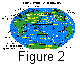However, since there are a number of cosmic-ray neutron monitors distributed over the land surface of the Earth (compare Figure 2 to see the situation in 1997), they may be used to obtain a(t) whereas g(t) can be obtained from satellite data.

### 2. Computational Method

In principle, one could use the ground level neutron monitor data as adjoint sources (compare Figure 2) and solve the adjoint form of the transport equation, applying the satellite spectra as boundary conditions. However, since many radiation components contribute to the response of a neutron monitor, an equal number of adjoint calculations would have to be calculated for each value of g. A simpler and more straightforward approach is to execute a forward calculation of the neutron monitor response for a range of values of g and interpolate among them. The value of a in the equation for the flux is determined by setting the integral flux above 100 MeV to unity for each value of g.

Boltzmann's equation is a continuity equation in phase space that is made up of the three space coordinates of Euclidean geometry, the kinetic energy, and the direction of motion of the particles:

Bi fi (x, E, W, t) =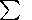j Qij

Bi = W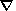+si + di -(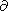/Ei)Si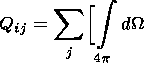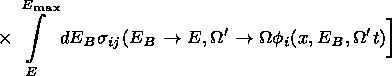di= (1-b2)1/2/tc b

where Bi is the Boltzmann operator; si is the absorption cross section for particles of type i; di is the decay probability per unit flight path of radioactive particles (such as muons or pions) of type i; Si is the stopping power for charged particles of type i (assumed to be zero for uncharged particles); fi(x,E,W,t) is the particle flux of type- i particles at location x, energy E, direction W, and time t; Qij is the "scattering-down" integral, the production rate of particles of type i with a direction W, an energy E at a location x, by collisions with nuclei or decay of type-j particles having a direction W' at a higher-energy EB; sij is the doubly differential inclusive cross section for the production of type- i particles with energy E, and a direction W from nuclear collisions or decay of type-j particles with an energy EB and a direction W'; bi is the speed of a particle of type i with respect to the speed of light ( = vi/c); ti is the mean life of a radioactive particle of type i in the rest frame; and c is the speed of light in vacuo.

To calculate the neutron monitor response, it is necessary to know the proportional contribution each and every component of the ground level flux makes to the total counting rate. We have these data as a result of the work of Hughes and Marsden . We need also to know the absolute values of the fluxes that correspond to a given counting rate, and we have these data as a result of our work with cosmic rays only for the Goose Bay monitor in Canada and the Thule monitor in Greenland. The Thule monitor is now out of service. The Deep River monitor, for which we also had calibration data, has been dismantled.

We can get around this problem by calculating what the response of the Goose Bay monitor would be at each of the locations of the other ground level neutron monitors and by obtaining the ratio of its response to that of the local monitor. The assumption is that the energy and particle-type response are the same as that of the Goose Bay monitor.

To do this, we need the counting rate in the hour before the event, which is used as the monitor counting rate background. This counting rate will be unaffected by the solar particle event with which we are concerned as it precedes it. This counting rate gives us the heliocentric potential, which is then used to calculate the response of the Goose Bay monitor at the location of the other monitors. The ratio between the theoretical Goose Bay monitor counting rate and the local monitor counting rate then calibrates the local monitor in terms of the Goose Bay monitor. The difference between the background counting rate and the actual counting rate as the event precedes is due to the solar particle event.

The ratio of the actual counting rate to the calculated counting rate produced by a unit flux above 100 MeV is used to give us the solar-particle flux in the atmosphere above the monitor's location, which is then used to calculate the ionization as a function of time and altitude. The ionization, or ion-pair production rate, is given as I, ion pairs per cm3 s of air at normal temperature and pressure (NTP).

There are a number of crucial assumptions in this treatment:

1. The satellite spectra are an adequate representation of the energy distribution of the local proton spectra with rigidities greater than the various zenithal and azimuthal cutoffs proper to that location.

2. The form of the satellite spectra determined by satellite detector measurements up to 700 MeV is valid at higher energies.

3. The increase in the counting rates above the background counting rate (determined by the counting rate an hour before the event) is due only to the incident solar particles above the static cutoffs.

As for the last assumption, the cutoffs may be reduced from that of the quiescent geomagnetic field as a result of changes in the solar wind. Some of the increase would therefore be due to cosmic rays rather than solar particles. That increase contributes only a very small overestimate of the resulting atmospheric ionization rate. The assumption that the form of the satellite spectra are correct locally, at the neutron monitor locations, is an inescapable consequence of the amount of available data.

### 3. Neutron Monitor Data

The neutron monitor stations that were in existence and had useful data during ground level event 42 (GLE 42, September 29-30, 1989) and which we used to obtain the necessary adjoint source data are listed in Table 1.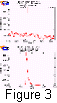These data and the associated counting rates (Figure 3) were obtained from WDC C2 for cosmic rays at Ibaraki University [WDC C2, 1998].

### 4. Satellite Particle Spectra

The satellite particle energy spectra used here were derived from data obtained by particle detectors aboard the GOES 7 satellite maintained by the NOAA Space Environment Center. These detectors measure the flux of energetic protons at geostationary orbit from energies of 600 keV to greater than 700 MeV (or momenta of 33 MeV c-1 to greater than 1300 MeV c-1 ) in 11 discrete channels. The observations of protons of greater than about 9 MeV (130 MeV c-1 ) are representative of those that would be obtained outside the magnetosphere in that protons of higher energy have full access to geostationary orbit (or alternatively, in that the geomagnetic cutoff at geostationary orbit has never been observed to exceed 9 MeV (130 MeV c-1 )).

The principal corrections that had been applied to these data were a correction for the HEPAD response to backward fluxes through it, and subtraction of the background counting rates in each channel due primarily to galactic cosmic rays, their progeny, and to a lesser extent, instrument noise.

### 5. Results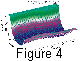Ionization calculations were carried out for 35 hours after the event, for elevations of sea level, to an elevation of 24,384 metres for each of the sites using a version of LUIN [O'Brien et al., 1996] designed for solar particle events. In Figure 4 we exhibit the hourly averaged distribution of ionization rate due to the solar particle event at the period of maximum intensity of the event at an atmospheric depth of 100 g cm-2.

The ionization rates due to GLE 42 exhibited here and in the following figures do not include the cosmic-ray "background" contribution, but are due to the solar particle event alone.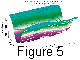It is interesting to note that the "knee" (the geomagnetic latitude above which the atmospheric cosmic-ray intensity remains constant) in the ionization rate distribution from GLE 42 (Figure 4) unlike the corresponding cosmic-ray distribution (compare Figure 5). The central "valley" resulting from the interaction of the incident particles is deeper and wider.

The cosmic-ray ionization rate distribution is narrower and not so deep as the GLE 42 ionization distribution because the cosmic-ray spectra are harder; that is, they have more particles at higher energies and are thus less effected by the geomagnetic field. The solar-particle ionization rate distribution is thus "U" shaped, while the cosmic-ray ionization distribution is "V" shaped.

The reason for the cosmic-ray knee is the declining efficiency of the primary cosmic-ray flux in producing secondary radiations in the atmosphere as one goes from high cutoffs at the equator to low cutoffs at the poles. At depths near and below the Pfotzer maximum the cosmic-ray flux is dominated by secondary cosmic-ray particles produced in the atmosphere. As one proceeds toward the poles the added primaries are at lower and lower energies with lower multiplicities, adding, for each increment of flux, a smaller and smaller addition to the total response of the atmosphere, in the case under discussion, ionization rate.

Solar particle spectra are less energetic and therefore less efficient at producing secondary radiations at all energies. Thus the added fluxes at low energies, added as one goes toward the poles, are more efficient relative to the secondary particle fluxes already present, adding secondaries and hence ionization rate. The high-energy cosmic-ray fluxes result in more atmospheric secondaries per unit flux than the solar particle fluxes; thus increases in the primary flux, even at low energies, have a larger effect in the latter case.

The cosmic-ray knee, therefore, does not result from some mysterious atmospheric cutoff. It is merely due to the changing balance between primary and secondary radiations in the atmosphere as a function of geomagnetic cutoff.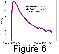The ionization as a function of time at an atmospheric depth of 100 g cm-2, over Durham, New Hampshire, in the United States, is shown in Figure 6. Note the rapid rise and decay. Twelve hours after maximum intensity, the ionization intensity has fallen by 1 and 1/2 orders of magnitude.

It is believed that any irregularities in the apparent solar-particle flux distributions are presumably due to the approximations we described and the existence of irregularities and/or gradients in the interplanetary medium as well as to variations in the access of these particles to the magnetosphere. It is demonstrable that significant perturbations of magnetospheric access can occur (at least at energies of the order of 500 MeV or less) as a result of the interference of interplanetary shocks and/or magnetic structures with the uniform propagation of solar energetic particles into the magnetosphere.

The ionization distribution from this large event has a maximum at the highest latitudes at an atmospheric depth of 100 g cm-2 of almost 2000  I.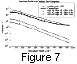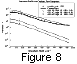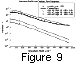In Figures 7, 8, and 9 we show the ionization profile at three sites from GLE 42 as a function of depth in the atmosphere at maximum intensity and then 12 and 24 hours afterward. The sites are Durham, New Hampshire; Sioux City, Iowa; and Palestine, Texas. The measured and calculated cosmic-ray intensity at these sites (which is the reason for the choice of these locations) is shown for comparison and also as an indication that the transport process at least does an adequate job in describing radiation propagation in the atmosphere.

### 6. Conclusions

A method of calculation of the response of the atmosphere to solar particle radiation has been described and applied to the resulting atmospheric ionization rate. Atmospheric ionization rate has been shown to have an impact on weather (Bago and Butler, in press). It is also a "dose-like" parameter and related to the radiation exposure of human populations, especially air crew. The results of these calculations have been compared, for illustrative purposes, with cosmic-ray ionization rates at a depth of 100 g cm-2, the so-called production layer. These comparisons clearly show the effect of the softer particle spectra associated with solar particle events, as compared with cosmic rays, resulting in a greater sensitivity to the geomagnetic field, and, unlike cosmic rays, the absence of a "knee" near 60o geomagnetic latitude.

#### Acknowledgment

The authors gratefully acknowledge the assistance of Takashi Watanabe, Acting Director of WDC C2 for cosmic rays, Ibaraki University, in supplying the world wide neutron monitor counting rates used as adjoint sources in the solar-particle flux calculations described in this paper.

### References

Bago, E. P., and C. J. Butler, The influence of cosmic rays on terrestrial clouds and global warming, Astron. Geophys., in press.

Ellison, D. C., and R. Ramaty, Shock acceleration of electrons and ions in solar flares, Astrophys. J., 298, 400, 1985.

Gosling, J. T., Coronal mass ejections: An overview, in Coronal Mass Ejections, Geophys. Monogr. 99, edited by N. Crooker, J. A. Joselyn, and J. Feynman, pp. 9-16, AGU, Washington, D.C., 1997.

Hughes, E. B., and P. L. Marsden, Response of a standard IGY neutron monitor, J. Geophys. Res., 71, 1435, 1966.

O'Brien, K., W. Friedberg, H. H. Sauer, and D. F Smart, Atmospheric cosmic rays and solar energetic particles at aircraft altitudes, Environ. Int., Suppl., 1, S9-S44, 1996.

WDC C2, http://env.sci.ibaraki.ac.jp, 1998.

Load files for printing and local use. This document was generated by TeXWeb (Win32, v.1.3) on July 8, 2001.